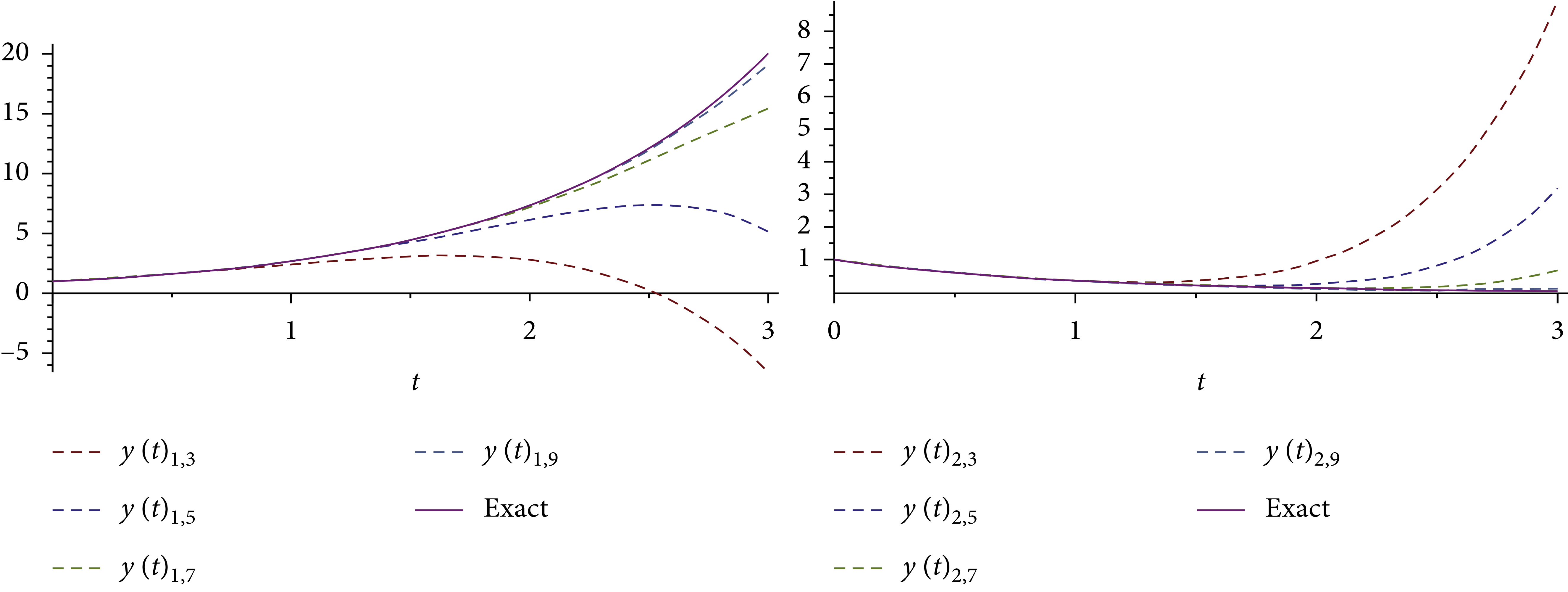Journal

# An Analytical Computational Algorithm for Solving a System of Multipantograph DDEs Using Laplace Variational Iteration Algorithm

By
Bahgat M.S.M.
Sebaq A.M.

In this research, an approximation symbolic algorithm is suggested to obtain an approximate solution of multipantograph system of type delay differential equations (DDEs) using a combination of Laplace transform and variational iteration algorithm (VIA). The corresponding convergence results are acquired, and an efficient algorithm for choosing a feasible Lagrange multiplier is designed in the solving process. The application of the Laplace variational iteration algorithm (LVIA) for the problems is clarified. With graphics and tables, LVIA approximates to a high degree of accuracy with a few numbers of iterates. Also, computational results of the considered examples imply that LVIA is accurate, simple, and appropriate for solving a system of multipantograph delay differential equations (SMPDDEs). © 2021 Mohamed S. M. Bahgat and A. M. Sebaq.